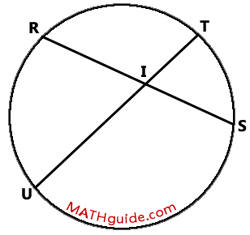Circle Properties
 Home > Lessons > Circle Properties Search | Updated December 2nd, 2015
Introduction
In this section of MATHguide, you will learn about the various sections there are related to circles and their properties.

 Circle Terminology This sections serves as the primer for learning about circles. It is necessary to know critical terms so that the relationships and equations can be understood. MATHguide has this lesson to teach you about the terminology.esson: Circle Terminology Central Angles and Arcs This section of MATHguide will inform you about angles at the center of circles and the arcs they intercept.esson: Central Angles and Arcs Inscribed Angles and Arcs An inscribed angle is an angle that has its vertex on a circle and its sides are chords of the same circle. This lesson will inform you of the relationship between these angles and the arcs they intercept of the circle.esson: Inscribed Angles and Arcs Chords There are two relationships related to chords, one for lengths of partial chords and the other for arcs and the angle subtended by those arcs. View this lesson to access explanations for both of those relationships.esson: Chord-Chord Relationships Secants This section deals with properties related to intersecting secant lines and the circles they also intersect. A picture of intersecting secant lines can be seen here.There are two different properties related to intersecting secant lines.esson: Secant-Secant Relationships